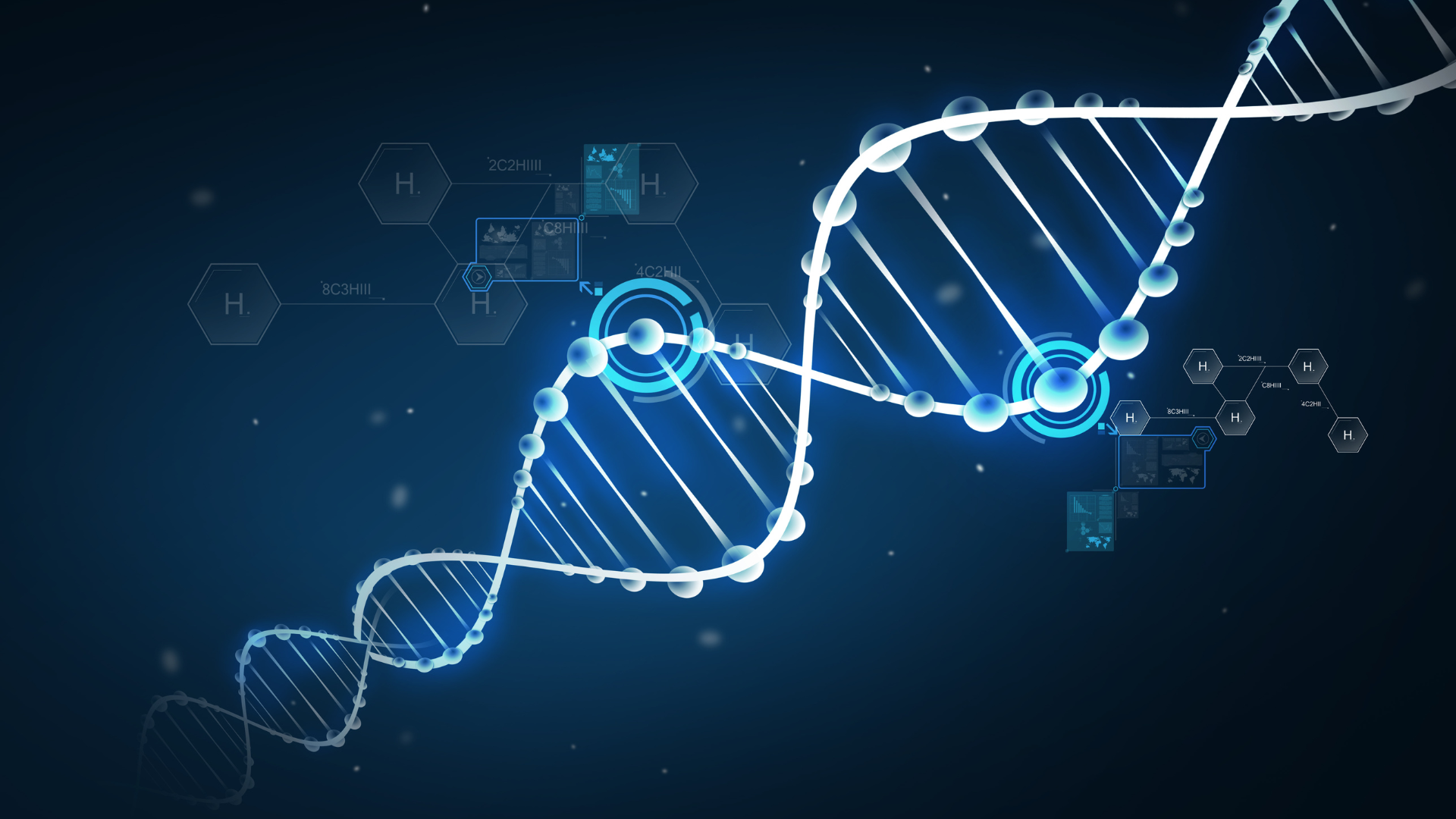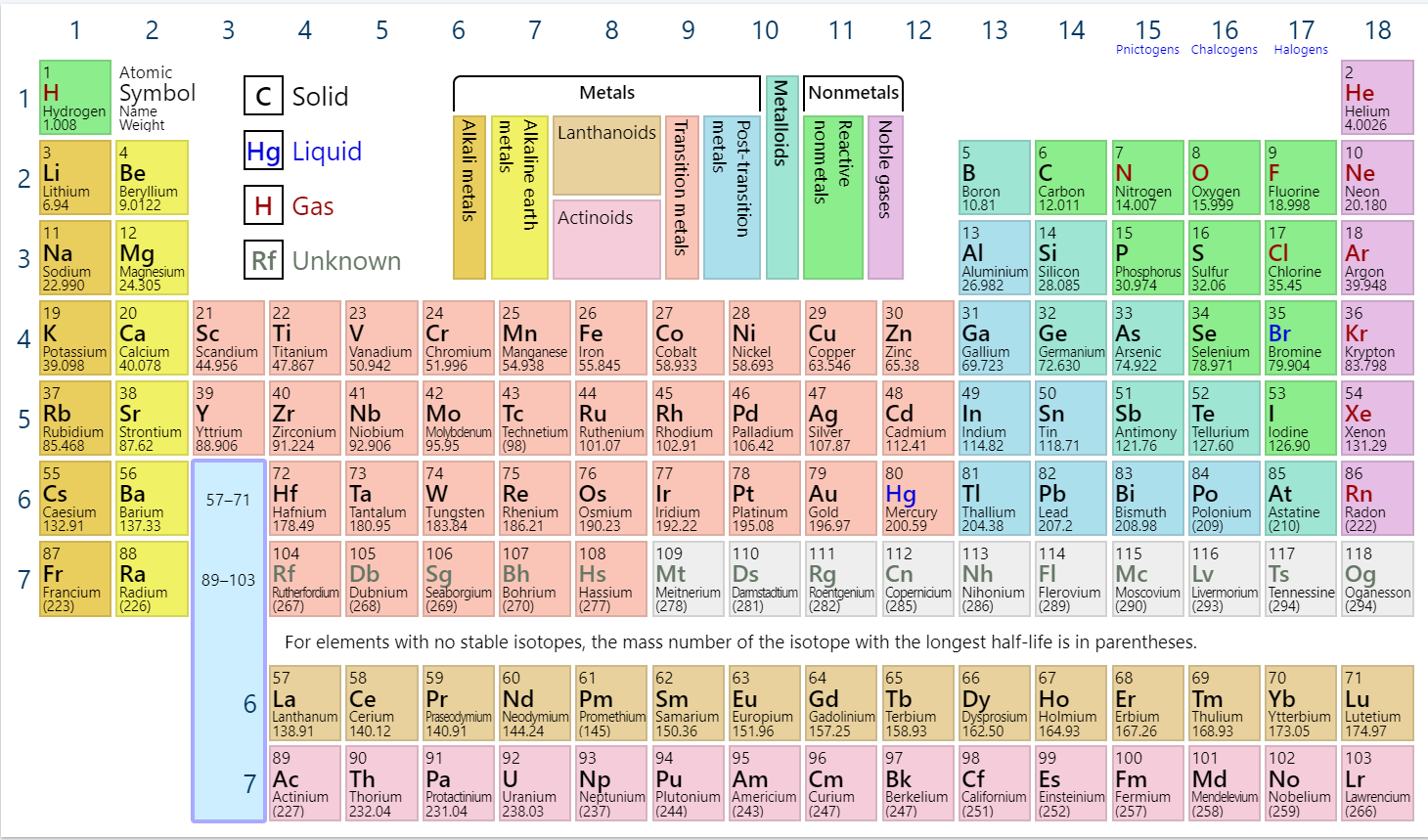Need help?

# What’s the Molar Mass of Hydrogen?

High school students around the world need to know the molar mass of elements. This is useful for a variety of science experiments.  Because testing and scientific experimentation involve measuring specific amounts of substances, the molar mass allows you to figure out how much you should weigh on your scale.  In this article, we’ll take you through everything you need to know about molar mass – and specifically the molar mass of hydrogen.#### What’s Molar Mass?

All types of scientific education require exact measurements of a substance. Things like chromium, titanium, iron and cobalt need to be measured extremely precisely in order to come to valid scientific conclusions. It’s for this reason molar mass is so important.

Molar Mass is defined as the ratio between the mass and the amount of substance (measured in moles) of any sample of a compound. This means whether you’re trying to figure out how much carbon, titanium, or cobalt you need for an experiment – understanding the molar mass is essential.

Take this example: say you need 4 moles of pure carbon for an experiment. You already know carbon has a molar mass of 12.01 g/mol. This means you can simply multiply this by 4 moles, and you’ll find out how much carbon you’ll need to weigh. You’ll need 48.04g of carbon.#### What’s the Molar Mass of Hydrogen?

The molar mass of hydrogen is 2.01568 g/mole. It can be calculated by multiplying the atomic weight of hydrogen (1.00784 atomic mass units) by 2. As hydrogen gas is formed of two hydrogen (H) molecules bound together (H2) – the molar mass can be calculated as 1.00784 x 2 = 2.01568 grams.

 Molar Mass: Hydrogen vs. Other Elements Element Chemical Formula Molar Mass Hydrogen H 1.00784 g/mol Sodium Na 22.98977 g/mol Nitrogen N 14.007 g/mol Chlorine Cl 35.45 g/mol Carbon C 12.011 g/mol Oxygen O 15.9994 g/mol Iodine I 126.9045 g/mol Zinc Zn 65.4 g/mol

#### Characteristics of Hydrogen

As hydrogen consists of only one proton, its atomic number is 1. The more protons an atom has in its nucleus, the higher the atomic number, and the element also becomes heavier.  This means that overall hydrogen is an extremely ‘light’ element.

It’s also very reactive. Because of this reactivity, atoms of atomic hydrogen (H) combine with each other to form molecules of two atoms (H2). This is how hydrogen molecules – H2 – are formed.

Molecular hydrogen (H2) can also react with many elements and compounds, but at room temperature, the reaction takes place at such a slow rate it's almost negligible. Because of this apparent inertness, the H2 molecule can be considered a very stable molecule at room temperature.

#### Uses of Hydrogen Molar Mass in Education

Knowing the molar mass of hydrogen will open up a world of opportunity in terms of hydrogen education. Students can understand, for instance, the process of hydrogen generation (electrolysis) with greater insight.

Why does a hydrogen generator (electrolyzer) actually produce 8 times more oxygen than hydrogen? The molar mass of 2 molecules of water (2 H20) is 18, and once the hydrogen generator splits the water into hydrogen and oxygen, the hydrogen (having a low atomic mass) only has a mass of 2 (H2), while the oxygen has a mass of 16 (O2). The result is for every 1 unit of hydrogen produced, 8 units of oxygen are created.

Interested in learning more about the possibilities of hydrogen education? Discover how the Horizon Hydrogen Grand Prix (H2GP) program can benefit students in a classroom near you.